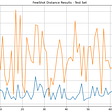# Time-Series Forecasting: How To Predict Future Data Using ARMA , ARIMA and SARIMA models.

ARIMA, ARMA and SARIMA are used for predict future data(Forecasting), that can be sale, stock price , no of visitors , supply data etc.

There are many models for data forecasting . but in this tutorial our main focus on discuss about these three models and how to do forecasting using these three models.

First let understand about ARMA, ARIMA and SARIMA models.

Before go on ARMA, ARIMA and SARIMA . let understand two basic model of forecasting.

1-Auto regression.

AR(p)

The value for “p” is called the order.

For example, an AR(1) would be a “first order auto regressive process.”

It mean forecasting of today depend on yesterday.

AR(2) would be a “second order auto regressive process.”

It mean forecasting of today depend on yesterday or day before yesterday.

AR(p) stands for the auto regression model, the p parameter is an integer that confirms how many lagged series are going to be used to forecast periods ahead.

Auto regression model predict future data base on previous data.

That previous data or value is called lags.

The AR(p) model is defined by the equation:

yt = δ + φ1yt-1 + φ2yt-2 + … + φpyt-1 + At

Where:

• yt-1, yt-2…yt-p are the past series values (lags),
• At is white noise (i.e. randomness) or error.
• and δ is defined by the following equation:

2- Moving average.

MA(q)

The value for “q” is called the order.

For example -

The 1st order moving average model, denoted by MA(1) is:

x t = μ + w t + θ 1 w t − 1 ,

The 2nd order moving average model, denoted by MA(2) is:

x t = μ + w t + θ 1 w t − 1 + θ 2w t − 2

The qth order moving average model, denoted by MA(q) is:

x t = μ + w t + θ 1 w t − 1 + θ 2w t − 2+…..+ + θ w t − q

MA(q) stands for moving average model, the q is the number of lagged forecast error terms in the prediction equation.

ARMA(p,q)

A-Auto.

R-Regression.

M-Moving.

A-Average.

ARMA is the combination of these twos Auto regression model + moving average model

This model take two parameter

1-p

2-q

AR(p) stands for the auto regressive model, the p parameter is an integer that confirms how many lagged series are going to be used to forecast periods ahead.

MA(q) stands for moving average model, the q is the number of lagged forecast error terms in the prediction equation.

AR(p) + MA(q) =ARMA(p,q).

ARIMA(p,d,q)

A-Auto.

R-Regression.

I-Integrated.

M-Moving.

A-Average.

ARIMA is a model that can be fitted to time series data to predict future points in the series.

We can split the ARIMA term into three terms, AR, I, MA:

AR(p) stands for the auto regressive model, the p parameter is an integer that confirms how many lagged series are going to be used to forecast periods ahead.

I(d) is the differencing part, the d parameter tells how many differencing orders are going to be used to make the series stationary.

MA(q) stands for moving average model, the q is the number of lagged forecast error terms in the prediction equation.

ARIMA model is best for predict forecasting, when the data is not seasonal.

SARIMA(p,d,r),(PDR)m

S-Seasonal.

A-Auto.

R-Regression.

I-Integrated.

M-Moving.

A-Average.

SARIMA is seasonal ARIMA and it is used with predict time series with seasonality.

p,d,r is the same of ARIMA

P,D,R is the analog version of p,d,r.

m — It is seasonal factor. which is the no of periods in a year seasonality repeated.

Let understand all about these model with the examples.

So, for better understanding about these three models, we consider Portland Oregon average monthly bus ridership dataset.

This is Uni-variate Dataset.

Save this csv file in your current directory.

Let begin with implement ARMA ,ARIMA OR SARIMA.

STEP 1-

`import pandas as pdimport numpy as npimport matplotlib.pyplot as plt%matplotlib inline`

Import pandas, numpy and matplotlib libraries.

STEP 2-

`data=pd.read_csv('portland.csv')data.head()`

Read the dataset from the file.

Dataset like this-

STEP 3-Check the shape of the data.

`data.shape`

115 rows with 2 feature.

STEP 4- Check the dataset contain null values or not.

`data.isnull().sum()`

Dataset doesn’t contains any null values.

STEP 5- Check the information about the data.

`data.info()`

Both columns is object type column contain no any null values.

STEP 6-Convert month (object type ) column into date-time or average ride column into integer for visualize the trend , seasonality , stationary etc.

`data['Month']=pd.to_datetime(data['Month'], errors='coerce', format='%Y-%m')data['Portland Oregon average monthly bus ridership (/100) January 1973 through June 1982, n=114']=data['Portland Oregon average monthly bus ridership (/100) January 1973 through June 1982, n=114'].astype(int)`

STEP 7- Rename column names.

`data.columns=['Month','avg monthly busride']`

STEP 8 -Now again check the information about the data.

`data.info()`

Now, Month is date-time type with 1 null value.

And average monthly bus ride now is in int type.

STEP 9- Drop null values from month column.

`data.dropna(subset = ["Month"], inplace=True)`

STEP 10- We will make the month column as a table’s index.

`data.set_index('Month',inplace=True)`

NOTE-Deal with time series data ,index of the table always date-time column with date time format for better visualization about the data.(it’s optional)

Now, Data like this-

STEP 11-Plot the data for finding time series components.

`data.plot()`

NOTE- We already know ARIMA , ARMA Model take assumption data is in stationary.

Because , Forecasting a stationary series is relatively easy and the forecasts are more reliable.

Stationary- mean or S.D does not change over the time.There is no trend and seasonality.

NON stationary- mean or S.D change over the time.There is trend and seasonality.

But above data follow some trend with seasonality.

Q-How to check whether data is stationary or not.

ANS-There are many methods to check whether data is stationary or not.

1-Using graph. we can find data is stationary or not using visualization in form of graph.

2-Using Some statistics test.

Recommended-statistics test show strong evidence. so use statistics test to check data is stationary or not with strong evidence.

For check data is stationary or not .we have DICKEY-FULLER test.

STEP 12- Check the data is stationary or not using DICKEY-FULLER test.

Ho- Fail to reject null hypothesis . it mean data is stationary.

H1- Reject the null hypothesis. it mean data is not stationary.

If p value is less than threshold value is 0.5 fail to reject the null hypothesis and it mean data is stationary.

If p value is more than threshold value 0.5. reject the null hypothesis accept alternative hypothesis.it mean data is not stationary.

`from statsmodels.tsa.stattools import adfullerx=data['avg monthly busride']result=adfuller(x)print("ADF Stataics ",result)print("p-value",result)print("critical values",result)if result<=0.05:    print("fail to reject null hypothese h1 , it mean data is stationary")else:    print("Reject the null hypotheise , it mean data is not stationary")`

Right there, clearly show p value is greater than 0.5 , so reject the null hypothesis and accept alternative hypothesis.

It mean data is not stationary.

There are many ways to convert non stationary data into stationary.

1–1st difference or 2nd. or 3 rd difference (most popular method).

2- logarithm difference.

3- Take a moving average with length as the seasonal window. This will smoother in series in the process.

ETC.

STEP 13-Convert non stationary data into stationary using 1st difference.

`data['avg monthly busride first difference']=data['avg monthly busride']-data['avg monthly busride'].shift(1)`

In 1st difference subtract current data from the previous one shift data.

If series will not be become stationary. we will perform second difference and so on until or unless data will not become stationary.

STEP 14- Now checking data is stationary or not using graphs.

`data['avg monthly busride first difference'].plot()`

Data look like stationary , mean or S.D is not changing over the time.

STEP 15-For strong evidence again , we are checking with DickeyFuller statistics test.

`x=data['avg monthly busride first difference'].dropna() result=adfuller(x)print("ADF Stataics ",result)print("p-value",result)print("critical values",result)if result<=0.05:    print("fail to reject null hypothese h1 , it mean data is stationary")else:    print("Reject the null hypotheise , it mean data is not stationary")`

Now, after perform 1st shift p value is less than 0.5 . so accept the null hypothesis and reject alternative hypothesis. it mean data is stationary.

Data become stationary , so do not need more differencing.

STEP 16- After performed all preprocessing and testing. time to implement ARMA ,ARIMA or SARIMA models.

First of all try with ARMA model.

Before implement ARMA model let understand some important terms.

We already known ARMA take two parameters (p,q)

Q-question is how to find out what value of p and q are best for our forecasting.

ANS- for this there are two functions .

1-ACF.

2-PACF.

Use Auto correlation function to find q value .

Use partially Auto correlation function to find p value.

Let see how these can be implement-

`from statsmodels.graphics.tsaplots import plot_acf,plot_pacfplot_acf(data['avg monthly busride first difference'].iloc[1:],lags=30)plot_pacf(data['avg monthly busride first difference'].iloc[1:],lags=30)`

Using these two functions. we selected p=2 and q=2.

STEP 17-Implement first ARMA model.

`from statsmodels.tsa.arima_model import ARMAmodel=ARMA(data['avg monthly busride'],order=(2,2))model_fit=model.fit()`

First import ARMA model

Initialize ARMA with p and q values order(2,2).

Fit the model.

STEP 18-Check the summary of the ARMA model.

`model_fit.summary()`

STEP 19-Compare ARMA’S predict value with the real value using graph-

`data['ARMA_forecast'] = model_fit.predict(start = 92, end= 114, dynamic= True)  data[['avg monthly busride', 'ARMA_forecast']].plot(figsize=(8, 5))`

We are predicting data from 92 to 114

92 is the starting point of prediction.

114 is the ending.

Prediction is looking good. but it is not form in seasonal.

We known if data is seasonal then SARIMA is best model for Forecasting.

STEP 20-Now , PREDICTION CHECK WITH ARIMA.

p=2

d=1(because we did only one difference to convert non stationary to stationary so we are considering it 1.

q=2

`from statsmodels.tsa.arima_model import ARIMAmodel1=ARIMA(data['avg monthly busride'],order=(2,1,2))model_fit1=model1.fit()data['forecast_ARIMA'] = model_fit1.predict(start = 92, end= 114, dynamic= True)  data[['avg monthly busride', 'forecast_ARIMA']].plot(figsize=(8, 5))`

Forecasting is very poor with ARIMA.

STEP 21- Check how SARIMA will perform on this seasonal data.

`import statsmodels.api as smmodel=sm.tsa.statespace.SARIMAX(data['avg monthly busride'],order=(2,1,2),seasonal_order=(2,1,2,6))result=model.fit()`

Apply SARIMA on same dataset with

order(2,1,2) same like ARIMA.

Seasonal_order(2,1,2,4) because it’s analog version of ARIMA’S p,d,q.but here P,D,Q represent seasonal order.

4 is seasonal factor. which is the no of periods in a year seasonality repeated.

In our dataset in a year 4 time pattern is repeating .so we have consider seasonal factor 4.

STEP 22-Compare predict data with real data.

`data['forcast_SARIMA_1']=result.predict(start=99, end=112, dynamic=True)data[['avg monthly busride','forcast_SARIMA_1']].plot(figsize=(20, 5))`

SARIMA model is working well. because data is seasonal.

So, we are try to predict future forecasting using SARIMA model.

STEP 23-

`import datetimefrom dateutil.relativedelta import relativedeltastart = datetime.datetime.strptime("1969-07-01", "%Y-%m-%d")date_list = [start + relativedelta(months=x) for x in range(0,12)]future_prediction = pd.DataFrame(index=date_list, columns= data.columns)data = pd.concat([data, future])`

Right here , we are creating new date time (future date time) for check what’s forecast will have with this future data.

And concatenation date time with real values.

STEP 24-Predict future forecasting using SARIMA.

`data['future_prediction']=result.predict(start=113, end=130, dynamic=True)data[['avg monthly busride','future_prediction']].plot(figsize=(10, 6))plt.grid(True)`
`data['future_prediction'] = result.predict(start = 113, end=130, dynamic= True)  data[['avg monthly busride', 'future_prediction']].ix[-20:].plot(figsize=(10, 6))`

Forecasting looking good.

--

--

--

## More from Puneet Singh

Data Science , Machine Learning , BlockChain Developer

Love podcasts or audiobooks? Learn on the go with our new app.

## Recommended from Medium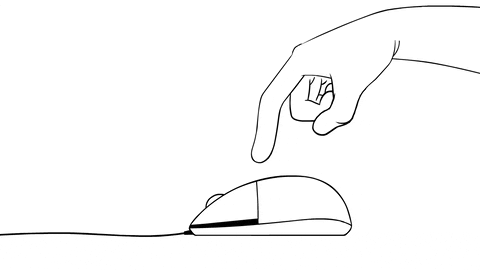## Twitter sentiment analysis: what does people say about Brazil’s ongoing pension reform?## Epidemic Modeling 102: All CoVID-19 models are wrong, but some are useful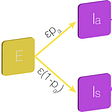## Starting guide to artificial intelligence part 2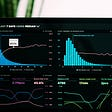## Fixing A Terrible Map (COMM 364)## Analytics and resilience, what we can learn from a pandemic## Reflections on reaching women over the phone in rural India## SRL Applications part2: SRL for (open) information extraction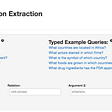## Puneet Singh

Data Science , Machine Learning , BlockChain Developer

## Classifying Flower through Sequential-API-Deep Learning.## Allstate Claims Severity — How severe is an insurance claim?## Microsoft Malware Kaggle challenge: Multiclass Classification and feature Enginnering.## Metric Learning for Steel Surface Classification — Part 2